Function Repository Resource:

# CycleLengthCounts

Count the number of cycles for all possible cycle lengths in a permutation

Contributed by: Wolfram Staff (original content by Sriram V. Pemmaraju and Steven S. Skiena)
 ResourceFunction["CycleLengthCounts"][p] returns a SparseArray of the number of cycles of each length in the permutation p.

## Details and Options

ResourceFunction["CycleLengthCounts"][p] returns a SparseArray of length n, where n=Length[p].
The list {λ1,λ2,} given by Normal is also known as permutation type.

## Examples

### Basic Examples (2)

Find the number of cycles of each length in a permutation:

 In:=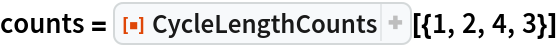Out=Use Normal to see the values:

 In:=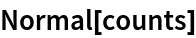Out=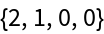The identity permutation of length n has n trivial cycles, that is, n 1-cycles:

 In:=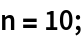In:=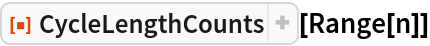Out=In:=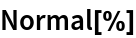Out=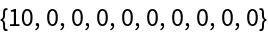Verify by counting cycles:

 In:=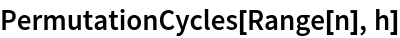Out=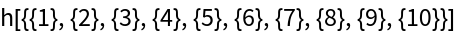### Properties and Relations (3)

A reverse of the even-length identity permutation of length n has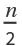2-cycles:

 In:=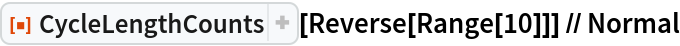Out=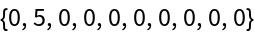The number of cycles in a permutation is the total of the cycle length counts:

 In:=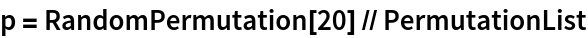Out=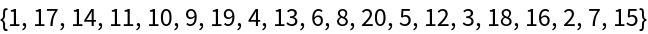In:=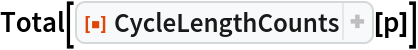Out=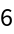In:=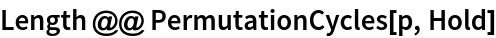Out=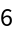The sum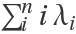is n:

 In:=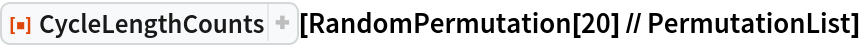Out=In:=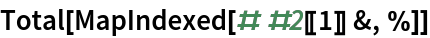Out=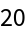## Version History

• 1.0.0 – 09 July 2020